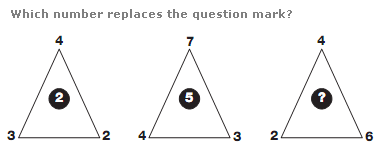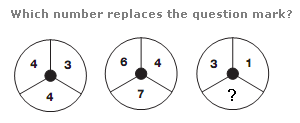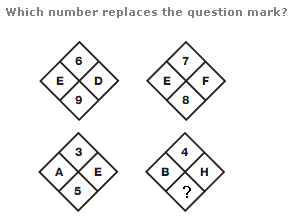# Puzzles - Number puzzles

### Exercise :: Number puzzlesAnswer : 8 Explanation : In each triangle, multiply the lower 2 numbers together and subtract the upper number to give the value in the centre.Answer : 10 Explanation : For each circle, add the left and right hand numbers together and put this total in the circle one place to the right.Answer : 7 Explanation : In each square, multiply the top and bottom numbers together to give a 2 digit result, and put the letters with the numerical values of each digit in the left and right hand spaces.